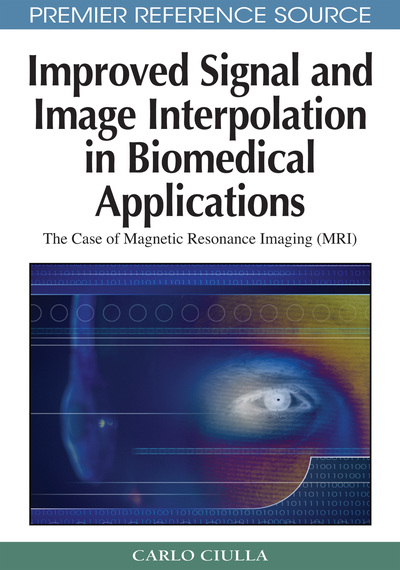# The Extension of the Theory to the Trivariate Linear Interpolation Function

Carlo Ciulla (Lane College, USA)
DOI: 10.4018/978-1-60566-202-2.ch010
Available
\$37.50
No Current Special Offers

## Abstract

The organization of this chapter is similar to that of Chapter VII where it was outlined the theoretical approach to the improvement of the bivariate linear interpolation function. The methodological approach calculates the Intensity-Curvature Functional on the basis of the two intensity-curvature terms calculated before and after interpolation. The roots of the polynomial system consisting of the three first order partial derivatives of the Intensity-Curvature Functional constitute the SRE. The formula that belongs to the unifying theory and that allows the calculation of the novel re-sampling locations for the trivariate linear interpolation function is in this chapter seen in equation (1). The novel re-sampling locations (xr0, yr0 , zr0) are obtained through equations (14), (15) and (16).
Chapter Preview
Top

## Methodology

To capture the variation across the voxel of both intensity and curvature of the function, the intensity-curvature term is defined as the product of the interpolation function times the sum of its second order partial derivatives: ∂2 (h (x, y, z))/δxδy, ∂2(h (x, y, z))/δxδz, and ∂2(h (x, y, z))/δyδz respectively. The intensity-curvature term is computed at the grid node and named Eo, and at the generic intra-pixel location and named EIN. The ratio Eo(x, y, z) / EIN(x, y, z) constitutes the Intensity-Curvature Functional: ΔE = ΔE(x, y, z). For ΔE, the first order partial derivatives are calculated with respect to x, y, and z. A polynomial system constituted by the first order partial derivatives of ΔE is thus obtained and its solution determines the set of points that constitute the Sub-pixel Efficacy Region. These sets of points are of the type (xsre, ysre, zsre) and they initiate the process of re-calculation of the interpolation function at each voxel.

Given a misplacement (x0, y0, z0), the misplacement used for signal interpolation is calculated based on the Sub-pixel Efficacy Region in order to improve the interpolation error and it consists of the novel re-sampling location (xr0, yr0, zr0). These novel re-sampling locations are derived through equation (1), which recalls equation (21) of Chapter VII.

{ h(xsre-xr0, ysre-yr0, zsre-zr0) * { [(∂2 (h(x, y, z))/∂x∂y) + (∂2 (h(x, y, z))/∂x∂z) +

## Complete Chapter List

Search this Book:
Reset